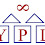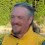# Moved. See https://slott56.github.io. All new content goes to the new site. This is a legacy, and will likely be dropped five years after the last post in Jan 2023.

## Thursday, June 6, 2013

### Obstinate Idiocy [Updated]

Once in a great while, you see someone engaging in Obstinate Idiocy.

Here's my recent example.

They're solving some kind of differential equation. Not sure why. Symptom 1 of Obstinate Idiocy is No Rational Justification. The explanation is often "that's not relevant, what's relevant is this other thing I want to focus on" or something equivalent to "never mind about that."

The equation is this:

$y + 3 \ln \lvert y \rvert = \frac{1}{3}(x-1)^3-\frac{11}{3}$

Pretty gnarly.

Apparently, they were so flummoxed by this that they immediately turned to Excel.  Really.  Excel.

Symptom 2 of Obstinate Idiocy is Random Tool Choice. Or perhaps Ineffective Tool Choice. A kind of weird, unthinking choice of tools.

Of course, Excel struggles with this sort of thing, since it appears to gnarly. I was told that there's an Excel Solver, but there was some problem with using it. It didn't scale, or it required some understanding of the shape of the equation or something.

Symptom 3 of Obstinate Idiocy is Seemingly Random Whining. It's random because there's no rational justification for what's going on and the tool was chosen apparently at random.

Ask a question like "why not use another tool?" and you don't get an answer. You get an argument about tool choice or the politics of the situation or "tool choice isn't the point" or some other dismissive non-answer.

Ask a question like "what are you really trying to do?" and you get user stories that make approximately no sense. We had to endure a long discussion on system-assigned surrogate keys as if that was somehow relevant to the graphing the equation shown above. See Symptom #1. There's just no reason for this. It's Very Important, but No One Else Can Understand The Reason Why.

How To Begin?

So, now we're at this weird impasse.

We have the obstinate idiot who won't discuss their tool choice. Somehow I'm supposed to sprinkle around some Faerie Dust and magically make Excel do something better or different than what it normally does. Indeed, I'm having trouble understanding any of the whining about Excel.

Clearly, they've never heard of MatLab or Mathematica or any commercial product that does this nicely. Apparently, they've never even seen the graph tool on Mac OS X which simply draws the graph with no effort of any kind on the part of the user.

Clearly, they've never seen Google and can't make it work.

They asked how a Pythonista would approach a problem this gnarly. I couldn't even properly understand that question, since they hadn't Googled anything and didn't really have a question that could be answered. As a Pythonista, I use Google. I wasn't sure how to approach an answer, since I couldn't really understand what their goal was or what their knowledge gap was.

Since their principal complaint was about Excel, asking a Python-related question didn't make much sense. Were they planning on dropping Excel? If so, why not use MatLab or Mathematica?

See Symptom 2. The tool choice was fixed. Other tools weren't on the table. If so, why ask about Python?

At this point, the place to begin seems to be this link: http://bit.ly/11usbtH And that's not going to be helpful with the Obstinate Idiot. They'll claim they already knew all of that, they just needed some additional or different help.

They specifically said they weren't going to use Python. Which raises the question "Why ask me anything, then?" To which there was no real answer, just sulking about me not being helpful.

Correct. I'm not being helpful. I can't figure out what the problem is. There's a gnarly formula and Excel somehow doesn't work in some optimal way. And database surrogate keys. And departmental politics.

## Did You Try This?

The equation simplifies to

$x = (3y + 9 \ln \lvert y \rvert + 11)^{\frac{1}{3}} + 1$

Which is really easy to graph. $$x=f(y)$$ is, of course, not the usual approach of $$y=f(x)$$.

Apparently, the Obstinate Idiot had not actually applied algebra to the equation. Nor had they ever conceived of graphing $$x=f(y)$$.

Which brings us to Symptom 4 of Obstinate Idiocy: Slow To Ask For Help.

And the variation on Symptom 1 of Obstinate Idiocy: Goal-Free Activity. By this I mean that the thrashing around with Excel and discussing Python was all just a long, drawn-out and utterly irrelevant side-bar from the real purpose, which apparently was to find something out related to a differential equation. It's still unclear what the equation is being used for and why the graph is helpful.

## Python Approach

First: Differential Equations are hard. Nothing makes them easy.

Interactive Python, however, can be of some help after you've taken the first steps with pencil and paper.

Here's a console log of something I did to help the Obstinate Idiot.

>>> import math
>>> import pprint
>>>
>>> def lde_1(y):
...     try:
...         x = (3*y+9*math.log(abs(y))+11)**(1/3)+1
...     except ValueError:
...         x = float("NaN")
...     return x
...
>>> def eval(y_lo=-15, y_hi=15, y_step=0.5, f_y=lde_1):
...     # Next smaller power of 2: prettier numbers. Less noise.
...     step_2 = 2**math.floor(math.log(y_step, 2))
...     for t in range(int((y_hi-y_lo) // step_2)):
...         y = y_lo + step_2*t
...         x = f_y(y)
...         yield( x, y )
...
>>> data1= list(eval())
>>> pprint.pprint(data1)


I'll leave out the dump of the data points. However, it's possible to see the asymptote at zero and the ranges where the results switch from real to complex numbers.

We can drill into the region around zero to see some details.

data2 = list(eval(-2, 2, .0625))
pprint.pprint(data2)


These are just numbers.  A picture is worth a thousand numbers.

We have lots of choices for graphic packages in Python. The point here, however, is that evaluating the gnarly equation required two preliminary steps that were far, far more important than choosing a graphic package.

1. Do some simple algebra.
2. Write a simple loop.

If output to Excel is somehow important, there's always this.

>>> import csv
>>> with open("data.csv","w") as target:
...    wtr= csv.writer(target)
...    wtr.writerows(data1)



That will produce a CSV that Excel will tolerate and display as an X-Y scatter plot.

A jupyter notebook with pyplot will knock out a picture directly, allowing visualization.

1.Easiest thing which is operating system independent and don't have to deal w/ fancy stuff like Mathematica is to

1) Bring up Wolfram Alpha

url: http://www.wolframalpha.com/

2) Enter in the following

y + 3*ln|y| = (1/3)( (x-1)^3 ) - (11/3)

2.Hi Steven, the images appear to be broken in the post - any chance you could re-insert them?

Thank you.

1.